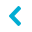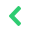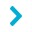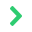Canvey Island, Essex, England
##### VM - Featured products
144MHz LFA Yagis

Low Noise LFA Yagis designed by G0KSC free to build for personal use.

Custom low-noise dish feeds
Custom low-noise dish feeds
G0KSC Custom Dish Feeds

Above installation @ HB9Q# 20el 16.2mtr Boom

##### G0KSC SC14420L 20el 144Mhz OWA Yagi Antenna with a 16.2 Metre Length BoomPremium commercial versions of this antenna at Innovantennas

This Yagi is optimised for 144 to 144.500Mz and is an EME Monster! It has a better G/T figure than most anything on the VE7BQH G/T table although it's design makes it narrow band. Direct 50 Ohm feed so non messy connectivity.

This is a field day/contest/EME antenna which will give excellent performance with veyr high average gain figures and best-in-class G/T figures too.

Dimensions in Metres

Element spacing:

• Ref =      0
• Driven = .554
• D1 =      .812
• D2 =      1.394
• D3 =      2.144
• D4 =      2.964
• D5 =      3.827
• D6 =      4.734
• D7 =      5.661
• D8 =      6.619
• D9 =      7.535
• D10=     8.539
• D11=     9.47
• D12=     10.449
• D13=     11.433
• D14=     12.438
• D15=     13.391
• D16=     14.378
• D17=     15.327
• D18=    16.20

Element sizes per element half:

• Ref =       .505
• Driven =   .498
• D1 =        .474
• D2 =        .461
• D3 =        .452
• D4 =        .447
• D5 =        .442
• D6 =        .437
• D7 =        .432
• D8 =        .429
• D9 =        .428
• D10=       .421
• D11=       .421
• D12=       .416
• D13=       .413
• D14=       .410
• D15=       .410
• D16=       .408
• D17=       .412
• D18=       .437

Performance figures @ 144.300Mhz:

• Froward Gain: 19.03dBi free space
• Front to Back: 25.64dB

Element diameter:

Each element is made out of single piece 8mm aluminum rod Each element half length needs to be doubled in order to gain your total element size. Please add 1mm to each end of each element if using tube instead of rod.An OWA does not hold a tight pattern. However, with a very good average gain figure, this antennagive excellent G/T performanceA Symmetrical, low-noise elevation plot tooSWR Plot nice and low.This antenna is 7.79W/L long. See how many Antennas on the VE7BQH list match this one for G/T?

Enjoy the antenna and please send photos!